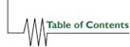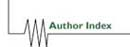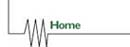Cray T3E High Performance Vectorized Libm Intrinsics

Neal Gaarder,
System Libraries Group
Cray Research, Inc.
655-F Lone Oak Drive
Eagan, Minnesota, USA

`nealg@cray.com```` ````www.cray.com```` ```

ABSTRACT:
This paper describes a new vectorized Math Library (libm) capability in Release 3.0 on the Cray T3E. These are select intrinsics, such as SQRT, EXP and POPCNT, which operate on a "vector" of arguments in memory and return a "vector" of results in memory. These vector intrinsics are typically 2-4 times faster than their scalar (one result per call) counterparts. Applications and performance are discussed.

KEYWORDS:
Performance, libm, libmfastv, Compilers, Optimization, Libraries, Vectorization, T3E

## Introduction

A new capability of Cray's Programming Environment 3.0 is optional "vectorization" of select intrinsic routines such as SQRT and POPCNT. Applications which currently spend a significant portion of the time computing math intrinsic functions can speed up significantly. One customer application ran twice as fast on the T3E with vectorization, although this is not typical.

Scalar intrinsics, such as SQRT and EXP, are functions that return one result per call. As one might expect, a "vectorized" intrinsic returns more than one result per call. On the MPP, these intrinsics operate on arrays ("vectors") in memory and return their results as arrays in memory. For example, a vectorized SQRT intrinsic processing an array of NARGS arguments, ARG_VEC, and returning an array of results, RSLT_VEC, might be

SQRT_V( NARGS, ARG_VEC, RLST_VEC).

Because they are called with an array of arguments, vector intrinsics can be optimized in ways that are not profitable or possible when given one argument. The vector intrinsics pipeline, unroll, prefetch, avoid calling overhead, and overlap more operations.

In many cases the remaining code is more efficient, as a function call inside a loop can trigger register spills and preclude other optimizations.

The vector routines themselves are typically 2-3 times faster than the equivalent scalar loop on the Cray T3E for loops with 100 iterations. Vector intrinsics can be as much as five times faster in certain cases.

## Vector intrinsics on the MPP

Over the years, Benchmarking's Tom Hewitt wrote many vector intrinsics for the T3D for internal use. These were collected into their library (bnchlib).

Silicon Graphics also developed vector math intrinsics.

Without compiler support, vector intrinsics had to be directly called by the user codes. This sometimes required a significant amount of recoding (as will be seen) so their application was limited. These routines were not generally available and not supported by normal channels. Also, while they are typically fully accurate over normal ranges, there were regions of lesser accuracy than libm and sometimes additional restrictions on the arguments.

With Cray's Programming Environment Release 3.0, the C and Fortran compilers automatically use vector intrinsics. They not only translate F90 vector syntax into vector calls, but also replace scalar intrinsic calls inside simple and complex loops. This can involve loop splitting, loop stripmining (processing a loop in chunks) and creating temporary arrays as needed for intermediate quantities.

## What will vectorize?

Both complex and simple loops will vectorize. The rules for vectorization are similar to those for Cray vector machines.

In PE 3.0 the following intrinsics vectorize:

• LOG (64 AND 32 BIT)
• COS (64 AND 32 BIT)
• COSS (64 AND 32 BIT) [ cos(x)+i*sin(x) ]
• EXP (64 AND 32 BIT)
• RANF (64 BIT) random number
• SIN (64 AND 32 BIT)
• SQRT (64 AND 32 BIT)
• 1/SQRT (64 AND 32 BIT) [New at Release 3.0]
• POPCNT (64 BIT) count of bits in a word

Note: LOG, COS, COSS, SIN, and 32 bit 1/SQRT and EXP require the -lmfastv link option (explained later) for significant savings.

end do

## Libm

The default library (libm) is built to meet the needs of the most exacting customers:

• full accuracy for all arguments;
• identical results whether scalar or vector;
• detect all special or invalid arguments;
• allow the entire range of arguments;
• good performance for short loops (30-60 iterations);

The default library libm contains a subset of the functions listed earlier. These are:

• SQRT(64 bit)
• SQRT(32 bit)
• 1/SQRT(64 bit)
• EXP(64 bit)
• RANF
• RANDOM_NUMBER
• POPCNT

## Libmfastv

A supplemental library was created to meet the needs of customers when they can tolerate slight inaccuracy, avoid special arguments and are dominated by somewhat longer loops (40 iterations or more). This library is named libmfastv. It contains all of the intrinsics listed earlier.

Those familiar with Bnchlib will see that many libmfastv intrinsics are derived from the Benchmarking routines: COS, SIN, COSS, LOG, EXP(32 bit), and 1/SQRT(32 bit).

## How is vectorization requested?

Vectorization is included among several optimizations when -O3 is specified to the C and Fortran 90 compilers. If -O3 causes optimizations that are not desired, vectorization may be specifically enabled, using the -Ovector3 option to Fortran 90, or the -hvector3 option to C.

Since libmfastv is optional, the user must specify the option -lmfastv when linking. It must precede any -lm option.

Examples of command line options:

## Performance of the Vector Intrinsics

It is difficult to characterize any vector intrinsic with a single number. We start with some general observations and move to more specific situations to illustrate. The performance improvements will vary with the situation.

The amount of cache residency is especially important to the comparison. We have tried to maximize it for these timings, as it seemed appropriate to minimize the impact of memory access time on the comparisons. This would correspond to the common case where an argument vector is computed and then operated on, all inside a fairly small kernel. Observed improvement undoubtedly will vary widely.

By taking multiple consecutive timings, and ignoring the first, data and instruction residency in the caches is maximized.

The first characteristic is known as the breakeven point. It is the number of loop iterations when the vector intrinsic first becomes as good as, or better than, the scalar version in a loop.

Special attention was given during development to making SQRT and EXP as fast as possible, as they are the most likely to be a significant factor in the performance of a broad range of user applications. For SQRT and EXP (64 bit), breakeven is under ten iterations. For the other functions, it varies widely, but 30-60 iterations is reasonable, especially for the libmfastv versions.

The following table shows the speedup factor for vector SQRT, varying the number of iterations in the loop. Speedup over 1 is an improvement. All tests were on a single PE of a 300 MHz T3E.

### y(1:n) = sqrt( x(1:n) )

Array Size Speedup Factor

27

2.5x

81

2.9x

243

3.8x

729

4.1x

### y(1:n) = exp( x(1:n) )

Array Size Speedup Factor

27

1.7x

81

2.4x

243

2.5x

729

2.5x

## Experience with vectorization

The experience in field test was limited. Most of the applications that we have data on did not use intrinsics much and thus profited minimally (0%` to 3%) from vectorization. However, one customer application ran twice as fast, a 100% speedup. It was described as a DNA code that used intrinsics heavily.

This code may have benefited from more than just the intrinsic speedups, since in many cases the restructuring allows other optimizations to occur.

Suppose an application spends a fraction i of run time in vectorizable intrinsics, and it spends i*v seconds on intrinsics after vectorizing. Then a speedup of 1/(1-i+i*v) should be seen. For example, assume a code spends 50% of the time in vectorizable intrinsics. If the average vectorization speedup is 2.0, the application will be 33% faster (1.33x). If the average vectorization speedup is 3.0, the application will be 50% faster (1.50x).

## Effective use of vectorization

Most users need only specify the command line options to get good results with vectorization. In some cases, a user may need a better result. This section discusses some advanced topics which might help. The topics are:

• Controlling vectorization with CDIR\$ directives
• Avoiding vectorization of short loops
• Avoiding temporary arrays
• Avoiding branches and ifs
The rules for what vectorizes are similar in spirit to the rules on the Cray vector machines. For example, recursions, pointers and vector dependencies can inhibit vectorization.

## Vectorization directives

In some cases, it is desirable to be able to control vectorization within a code. Directives have been provided to do this. They are CDIR\$ or !DIR\$ in Fortran 90 or #pragma _cri in the C language.

• CDIR\$ VECTOR : begin vectorization
• CDIR\$ NOVECTOR : end vectorization
• CDIR\$ NEXTSCALAR : turn off vectorization for next loop
• CDIR\$ IVDEP : ignore vector dependencies in next loop

Multiple calls to RANF within a loop will not normally vectorize because the sequence of random numbers will not have the same order as with the scalar loop. If this is acceptable, use CDIR\$ IVDEP to make the compiler vectorize as shown below.

##### CDIR\$ IVDEP !! vectorize next loop, if possible do j=1,n b=ranf() a=2*ranf()+b end do

In some cases, it may not be desirable to vectorize a particular loop, perhaps because it is too short to do profitably. Use CDIR\$ NEXTSCALAR to inhibit vectorization of a single loop as shown below.

##### CDIR\$ NEXTSCALAR !! don't vectorize next loop do j=1,2 a(j)=sqrt(b(j)) end do

CDIR\$ directives will not inhibit vectorization of Fortran 90 array syntax when the vectorization option is specified.

In some cases, it may be desirable to vectorize only one section in a code. For this, place a CDIR\$ NOVECTOR at the beginning of the code, a CDIR\$ VECTOR in front of the loop, and a CDIR\$ NOVECTOR after the loop, as shown below.

## Short loops

As explained earlier, for every function there is a breakeven point where the additional overhead of vectorization outweighs the efficiencies. For libm routines like SQRT and 64 bit EXP this is typically 10-30 iterations. For libmfastv routines it is typically 30-50 iterations.

Currently, the compiler does not consider the loop count before vectorizing.

Some codes will have many short loops and it may pay to selectively vectorize only the sections with long loops. This can be done with NOVECTOR and VECTOR directives.

Over the years Cray has studied the loop characteristics of its customers. A large fraction of loops fall in the 20-250 class. On the MPP, even codes from vector machines with very long loops tend to use much smaller loops as the work is divided among hundreds of PEs. For this reason, Cray has devoted much effort to reducing the overhead of the most frequently used intrinsics.

## Long loops

Loops over 100 iterations generally vectorize well. If a temporary array is created, the compiler will process the loop in "strips" of up to 256 iterations. This is called stripmining. If the temporary array can be avoided, that is best. Otherwise an array of size n can be defined to hold the temporary data if that is all that prevents full vectorization.

## Avoiding temporary arrays

The vector intrinsics do not need to have the data in the first level cache for near peak performance, but having as much data in second level cache will improve performance. Temporary arrays increase the probability of cache conflicts and so should be avoided.

One of the most efficient techniques uses the same array for result and argument. For example, y(1:n) = sqrt( y(1:n) ).

Sometimes the compiler needs to allocate a temporary array to hold the argument and/or the result of a vector intrinsic. Examples are when an intrinsic is called with an expression as an argument, or the argument and/or result are scalars in the loop. Such a loop might be as follows:

##### do j=1,n scalar = max( 0.0, arg_vector(j) ) result_vector(j) = sqrt( scalar ) end do

Such a loop can be vectorized by creating a temporary array to hold the scalar computation as follows:

##### do j=1,n temp_vector(j) = max( 0.0, arg_vector(j) ) end do call sqrt_vector( n, temp_vector, result_vector )

The temporary array can be avoided by assigning the argument to result_vector (the compiler may do this) as follows:

##### do j=1,n result_vector(j) = max( 0.0, arg_vector(j) ) result_vector(j) = sqrt( result_vector(j) ) end do

In this form, SQRT has shown up to 5x speedup for larger loops, due to reduced cache conflict, versus 4.5x speedup with different argument and result arrays.

## Avoiding branches

In general it is good practice to avoid branches on RISC machines, such as the T3E. If a call to an intrinsic only occurs when some condition is met, vectorization is inhibited. For example, the following loop will not vectorize.

##### do j=1,n if( x(j) .gt. 0) then y(j)= sqrt( x(j) ) else y(j)= 0 end if end do

Once the call is made unconditional, this loop vectorizes. Even though a few more arguments may be processed, the great savings of vectorization will offset the cost. The following loop will vectorize.

## Conclusions

The vectorized intrinsic feature in Release 3.0 can significantly improve the performance of select applications, those which now spend a significant portion of their time in loops computing intrinsic functions such as SQRT and EXP. This once required changes to the user code, but with Cray Programming Environment 3.0 codes can be optimized with command line options. Optional vectorization directives can be inserted in the code for finer control.

Loops dominated by vectorizable intrinsics and with over 100 iterations will typically be two to three times faster with vectorization. Intrinsic intensive applications can be up to twice as fast, but the speedup varies with the application.

## Author Biography

Neal Gaarder develops Math Library (libm) software for Cray Research, a Silicon Graphics company. He developed the vector intrinsic library routines discussed here.`nealg@cray.com```` ````www.cray.com```` ```

```Neal GaarderTable of Contents | Author Index | CUG Home Page | Home ```